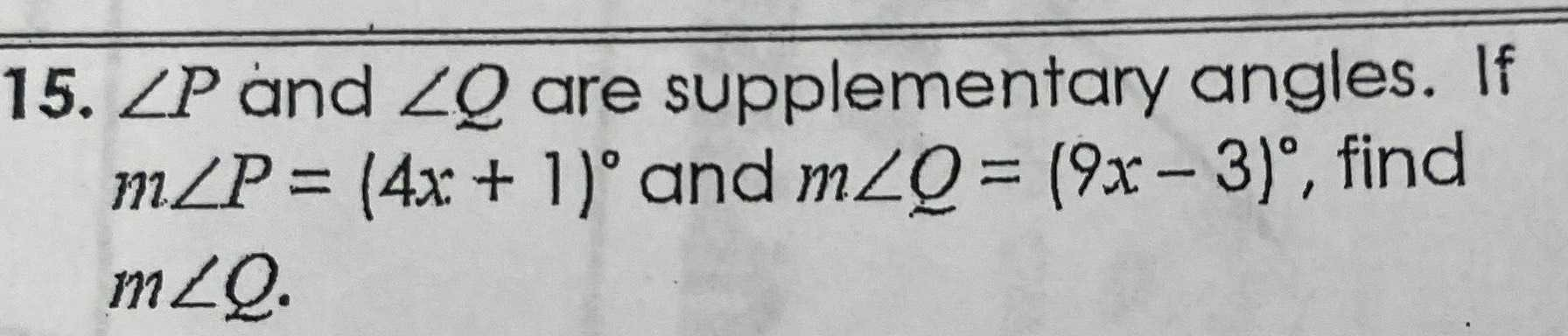### ¿Todavía tienes preguntas de matemáticas?

Pregunte a nuestros tutores expertos
Algebra
Pregunta15. $$\angle P$$ and $$\angle Q$$ are supplementary angles. If $$m \angle P = ( 4 x + 1 ) ^ { \circ }$$ and $$m \angle Q = ( 9 x - 3 ) ^ { \circ }$$ , find $$m \angle Q$$ .